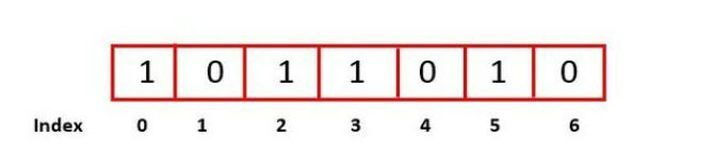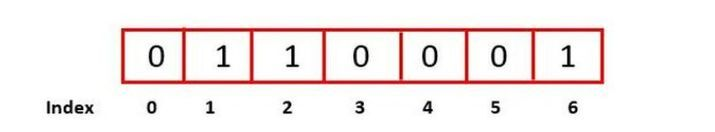# Check score of given binary string

The sequence of bytes is called a binary string and it holds the binary value. A binary score is normally presented on a range from 0 to 1 where 1 is reserved for the perfect model. In the given binary string, If the element is found to be 1 then it will calculate as the score and increment the count sum.

Let’s take an example of a binary score −

The given binary string is 1011010.In the above figure, the number 1 is present in the index- 0, 2, 3, and 5.

Therefore, the total score is 4 because there are a total of 4 indexes which having a binary score of 1.

The given binary string is 0110001.In the above figure, the number 1 is present in the index- 1, 2, and 6.

Therefore, the total score is 3 because there are a total of 3 indexes which having a binary score of 1.

In this article, we will find the score of the given binary string.

## Syntax

The following syntax used in the program

string_name.length()

## Parameters

• string_name

• length() − This function calculates the length of the string in terms of bytes.

## Algorithm

• We will start the program with a header file named ‘iostream’.

• Starting with the main function, we will declare a string variable "binary_str" and initialize it with a binary string. Also, initialize the variables for the count and store it with the value 0.

• We will create a for loop variable to set it as the counter based on the length of the binary string.

• Inside the for loop, we will use if-statement to check the i-th character of the binary_str is equivalent to 1. If the i-th character of the binary string is equivalent to 1 then the count will be incremented. Once the for loop is completed we will have the final count that will act as the score of the given binary string.

• After the loop is complete, we simply print the message as “The binary score of given number is:” followed by the count value.

## Example 1

In this program, we will implement the score of a binary string by using for loop. (Hint- If the index position of the string is found to be 1 then it will count as the score)

#include <iostream>
using namespace std;
int main() {
string binary_str = "101110101001";
int count = 0;
for( int i = 0; i <= binary_str.length(); i++ ) {
if( binary_str[i] == '1' )
{
count++;
}
}
cout<<"The binary score of given number is:\t"<<count;
return 0;
}


## Output

The binary score of given number is:	7


## Example 2

In this program, we will implement the score of a binary string by using a while loop. (Hint- If the index position in the string is found to be 1 then it will count as the score)

#include<iostream>
using namespace std;
int main() {
string binary_str = "1001";
int count = 0;
int i = 0;
while( i <= binary_str.length() ) {
if(binary_str[i] == '1') {
count++;
}
i++;
}
cout<<"The binary score of given number is:\t"<<count;
return 0;
}


## Output

The binary score of given number is:	2


## Conclusion

We explored the concept of binary string score and saw how length is useful to count the score of a given binary string. Generally, the computer understands only two numbers- 0 and 1 to perform various functions using them. For example- 0 and 1 are important for any IOT device.

Updated on: 20-Apr-2023

142 Views